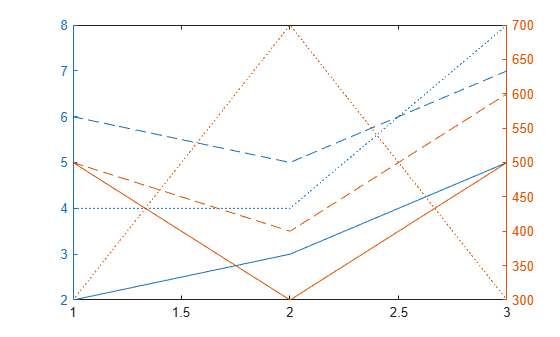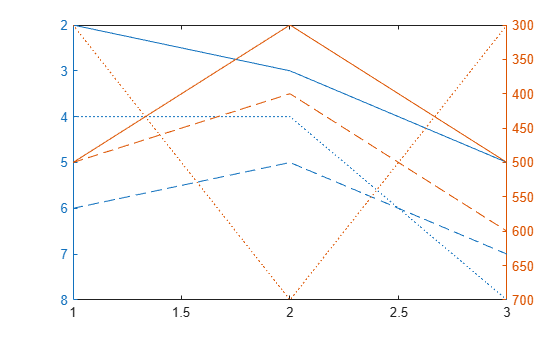Documentation

Modify Properties of Charts with Two y-Axes

The yyaxis function creates an Axes object with a y-axis on the left and right sides. Axes properties related to the y-axis have two values. However, MATLAB® gives access only to the value for the active side. For example, if the left side is active, then the YDir property of the Axes object contains the direction for the left y-axis. Similarly, if the right side is active, then the YDir property contains the direction for the right y-axis. An exception is that the YAxis property contains an array of two ruler objects (one for each y-axis).

You can change the appearance and behavior of a particular y-axis in either of these ways:

• Set the active side, and then change property values for the Axes object.

• Access the ruler objects through the YAxis property of the Axes object, and then change property values for the ruler object.

Change Axes Properties

Modify properties of a chart with two y-axes by setting Axes properties.

Create a chart with two y-axes and plot data.

x = [1 2 3];
y1 = [2 6 4; 3 5 4; 5 7 8];
y2 = 100*[5 5 3; 3 4 7; 5 6 3];
figure
yyaxis left
plot(x,y1)
yyaxis right
plot(x,y2)Reverse the direction of increasing values along each y-axis. Use yyaxis left to activate the left side and set the direction for the left y-axis. Similarly, use yyaxis right to activate the right side. Then, set the direction for the right y-axis.

ax = gca;
yyaxis left
ax.YDir = 'reverse';
yyaxis right
ax.YDir = 'reverse';Change Ruler Properties

Modify properties of a chart with two y-axes by setting ruler properties.

Create a chart with two y-axes and plot data.

x = [1 2 3];
y1 = [2 6 4; 3 5 4; 5 7 8];
y2 = 100*[5 5 3; 3 4 7; 5 6 3];
figure
yyaxis left
plot(x,y1)
yyaxis right
plot(x,y2)Reverse the direction of increasing values along each y-axis by setting properties of the ruler object associated with each axis. Use ax.YAxis(1) to refer to the ruler for the left side and ax.YAxis(2) to refer to the ruler for the right side.

ax = gca;
ax.YAxis(1).Direction = 'reverse';
ax.YAxis(2).Direction = 'reverse';Specify Colors Using Default Color Order

Specify the colors for a chart with two y-axes by changing the default axes color order.

Create a figure. Define two RGB color values, one for the left side and one for the right side. Change the default axes color order to these two colors before creating the axes. Set the default value at the figure level so that the new colors affect only axes that are children of the figure fig. The new colors do not affect axes in other figures. Then create the chart.

fig = figure;
left_color = [.5 .5 0];
right_color = [0 .5 .5];
set(fig,'defaultAxesColorOrder',[left_color; right_color]);

y = [1 2 3; 4 5 6];
yyaxis left
plot(y)

z = [6 5 4; 3 2 1];
yyaxis right
plot(z)